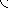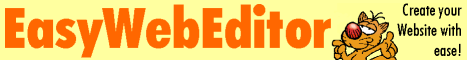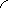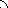Want a better software to manage your Web site more quickly and efficently? Click here, and start saving time today!

Create both Websites and CD DVD product catalogs with a single professional tool: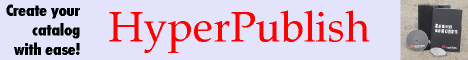This easy to use, highly functional Scientific Calculator is a must for science students. It includes triangle calculators, vector calculators, shape calculators, statistical calculators, half-life calculators, molar mass calculators, measurement converters and extensive data reference systems including 100 constants, 3000 compounds, 500 formulas, 400 symbols, ions, material properties, acids and bases, periodic tables and flow charts.

File size: 15370 Kb

WARNING: While we do our best to screen each file reviewed on CuteApps, we always suggest to scan the files with an antivirus before installing them (better safe than sorry).
Also, the download link was working at the time of the inclusion in this archive and when we did the last test; it is provided by the software house and sometimes can be -temporarily- offline or so.

## This easy to use calculator is a must for science students. It includes triangle calculators, vector calculators, shape calculators, statistical calculators, measurement converters, 3000 compounds, 100 constants, 500 formula and 400 symbols.

(Quickly and easily perform calculations with extensive science tools)This is the download page for DPLS Scientific Calculator - This highly functional Scientific Calculator is a must for science students. The main calculator has over 40 functions while being very easy to operate. The formula of a compound can be entered into the calculator and it will determine the molecular mass. The calculator output can be recorded in a electronic note pad in decimals, scientific notation or engineering notation. Included are an extensive number of science tools such as triangle calculators, vector calculators, shape calculators, kinematic calculators, half-life calculators, maths calculators, gas laws calculators, statistical calculators, molar mass calculators, pH calculators and one of the most extensive measurement converters available. Over 100 constants can be called to list their numerical value and uncertainty value. Included are over 500 formulas and equations for physics, chemistry, maths, trigonometry and statistics. It lists over 400 science, maths and statistics symbols. It lists the name, formula and molecular weight of over 3000 compounds. It contains many other science data reference systems such as SI units, derived quantities, maths laws, atomic structures, ions, homologous series, frequencies, shapes, angle types, material properties, acids, bases, conjugate pairs, ecological groups and biomes. The system contains extensive lists of piping and process symbols and electrical symbols, and it has interactive flowcharts for mechanical and electrical units. It contains 9 colour coded periodic tables and lists over 30 types of property data for each element. It contains full electron configuration of the elements, radiation types and quantum numbers. A glossary system contains the phonetic alphabet, science and medical fields, and acronyms and abbreviations used in science. The calculator also contains an event timer, yearly calendar and world times system. A help system explains operation, and a search system can be used to quickly locate data reference systems and science tools.